# Matrix

14 replies to this topic

### #1Chapas

Chapas

Newbie

•• Members
•• 19 posts

• Calculators:
Casio 9750, CP300

Posted 04 December 2010 - 05:00 PM

Hi guys.

I want to know how to solve a matrix like this one:I know its possible because i already do solve it, but now my CP returns a Argument type...

How can i do it ?

Best regards [[]]

### #2Chapas

Chapas

Newbie

•• Members
•• 19 posts

• Calculators:
Casio 9750, CP300

Posted 06 December 2010 - 01:45 AM

18 views and nobody knows how to solve it with classpad?

hugz [[]]

### #3pan.gejt

pan.gejt

Casio Freak

•• Members
•• 232 posts
• Gender:Male
• Location:CZ

• Calculators:
.

Posted 06 December 2010 - 04:41 PM

I found one solution only. I propose to solve this equation step by step. I rewrite the equation as A*B+C=D

1. multiply matrix A and matrix B and save the result as E (or anything else, depends on your priorities)
2. add matrix E and matrix C. This operation gives you system of 2 equations
3. use "solve" command for solving 2 equations (page 2.8.43 of user's guide for OS 3.05)

edited: TI-Nspire CAS allows quicker finding the solution. I used following syntax: solve(a*b+c=d,x,y). It gave me immediately the result x = -3 and y = 1.

Edited by pan.gejt, 06 December 2010 - 05:00 PM.

### #4300+Fan

300+Fan

Newbie

•• Members
•• 4 posts

• Calculators:
300+
OS 3.06

Posted 11 December 2010 - 12:19 AM

1. A*B (exe)
2. D-ans (exe)

solution:
x= -3
y= 1

### #5Guest_Classpad Warrior_*

•• Guests

Posted 11 December 2010 - 02:03 AM

If you guys get one for y, either there is something seriously wrong with your calculators or how you operate them. The answers are x=-3 and y=0.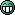### #6Guest_JBB_*

Guest_JBB_*
•• Guests

Posted 11 December 2010 - 02:17 AM

If you guys get one for y, either there is something seriously wrong with your calculators or how you operate them. The answers are x=-3 and y=0.After solveing the problem by hand, and by defining the matrices and using "Solve" on the TI89, I agree completely with Classpad Warrior. Multiplying the first two matrices, gives a two by one matrix with entries of 10 and 8 from the top down. Then adding the third matrix to this answer gives a two by one matrix with the top entry being 10+x and the bottom entry being 8+y. Setting that equal to the two by one matrix on the right side means that 10 +x must equal 7 and 8+y must equal 8 in which case, the answers must be x=- 3 and y=0 as Classpad warrior suggests. Note: It's easy to get into trouble using the nspire cas because it is so complicated to use. That thing is a real American piece of shit.

### #7300+Fan

300+Fan

Newbie

•• Members
•• 4 posts

• Calculators:
300+
OS 3.06

Posted 11 December 2010 - 05:04 AM

10
8 ?

A:
22
12

B:
3
2

A*B =
2*3+2*2=10
1*3+2*2= 7

or am i wrong ?

### #8pan.gejt

pan.gejt

Casio Freak

•• Members
•• 232 posts
• Gender:Male
• Location:CZ

• Calculators:
.

Posted 11 December 2010 - 06:48 AM

Classpad Warrior, JBB: you are wrong. How do you multiplicate the matrices?
300+Fan: you are right. The result is really 10 and 7.

### #9Guest_Casio warrior_*

Guest_Casio warrior_*
•• Guests

Posted 11 December 2010 - 06:04 PM

You are correct. I wrote the problem down incorrectly. Sorry about that.

### #10MicroPro

MicroPro

Casio Overlord

•• Deputy
•• 640 posts
• Gender:Male
• Location:Iran

• Calculators:

Posted 12 December 2010 - 05:58 AM

The matrices in classpad are designed to be fast and memory-saving. So equations can't be put in them. And the classpad's solve function expects lists to be passed in, not matrices. But this doesn't mean that you can't solve it in one line:
Define this function:
define solveMat(A,B,V)=solve(matToList(A-B,1),V)
Usage (you can use 2D keypad to enter matrices instead of what i wrote here):
solveMat( [[2,2],[1,2]]*[,]+[,[y]] , [,] , {x,y} )

Edited by MicroPro, 12 December 2010 - 06:07 AM.

### #11Chapas

Chapas

Newbie

•• Members
•• 19 posts

• Calculators:
Casio 9750, CP300

Posted 13 December 2010 - 02:29 AM

Micro PRO i didn't understood why you say to define the function?Could you explain better?

MicroPro:
"define solveMat(A,B,V)=solve(matToList(A-B,1),V)
Usage (you can use 2D keypad to enter matrices instead of what i wrote here)
solveMat( [[2,2],[1,2]]*[,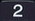2' />]+[,[y]] , [,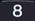8' />] , {x,y} )"

### #12MicroPro

MicroPro

Casio Overlord

•• Deputy
•• 640 posts
• Gender:Male
• Location:Iran

• Calculators:

Posted 13 December 2010 - 08:52 AM

That way you don't need to do the solve in multiple steps.
It can be done in only one line of calculation.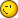Edited by MicroPro, 13 December 2010 - 08:55 AM.

### #13Guest_Mauritz_*

Guest_Mauritz_*
•• Guests

Posted 15 May 2011 - 05:04 PM

When I do it by hand i get that the product of the first two matrices is [10 1] (but as a column matrix), and the final answer is, as Nspire did answer, x=-3, y=1.
One could enter the matrixes directly into the solve function of Nspire.

And, I have owned both a classpad, and an Nspire, and I definitely prefer the Nspire.

By the way, is it now possible to define a functions with for, if , local variables and so on, on the classpad?

After solveing the problem by hand, and by defining the matrices and using "Solve" on the TI89, I agree completely with Classpad Warrior. Multiplying the first two matrices, gives a two by one matrix with entries of 10 and 8 from the top down. Then adding the third matrix to this answer gives a two by one matrix with the top entry being 10+x and the bottom entry being 8+y. Setting that equal to the two by one matrix on the right side means that 10 +x must equal 7 and 8+y must equal 8 in which case, the answers must be x=- 3 and y=0 as Classpad warrior suggests. Note: It's easy to get into trouble using the nspire cas because it is so complicated to use. That thing is a real American piece of shit.

### #14MicroPro

MicroPro

Casio Overlord

•• Deputy
•• 640 posts
• Gender:Male
• Location:Iran

• Calculators:

Posted 04 June 2011 - 08:17 AM

The fact that ClassPad's solve function doesn't accept matrices as input is a technical limitation of ClassPad's expression evaluator implementation. ClassPad's CAS is able to solve those equations. Just you need some tricks like solve(matToList(A-B,1),V) to express your inputs in a way CP CAS understands.

And yes I think CASIO people should improve the solve function so that it directly accepts matrices.

By the way, is it now possible to define a functions with for, if , local variables and so on, on the classpad?

Almost yes, in OS 3 you can run normal programs from the Main interface.Edited by MicroPro, 05 June 2011 - 08:54 AM.

### #15sma4t

sma4t

Newbie

•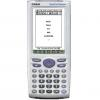• Members
•• 2 posts

• Calculators:
TI-89 Titanium

Posted 07 November 2018 - 10:49 PM

Thanks for suggesting the UDF, MicroPro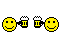The matrices in classpad are designed to be fast and memory-saving. So equations can't be put in them. And the classpad's solve function expects lists to be passed in, not matrices. But this doesn't mean that you can't solve it in one line:
Define this function:
define solveMat(A,B,V)=solve(matToList(A-B,1),V)
Usage (you can use 2D keypad to enter matrices instead of what i wrote here):
solveMat( [[2,2],[1,2]]*[,]+[,[y]] , [,] , {x,y} )

#### 0 user(s) are reading this topic

0 members, 0 guests, 0 anonymous users